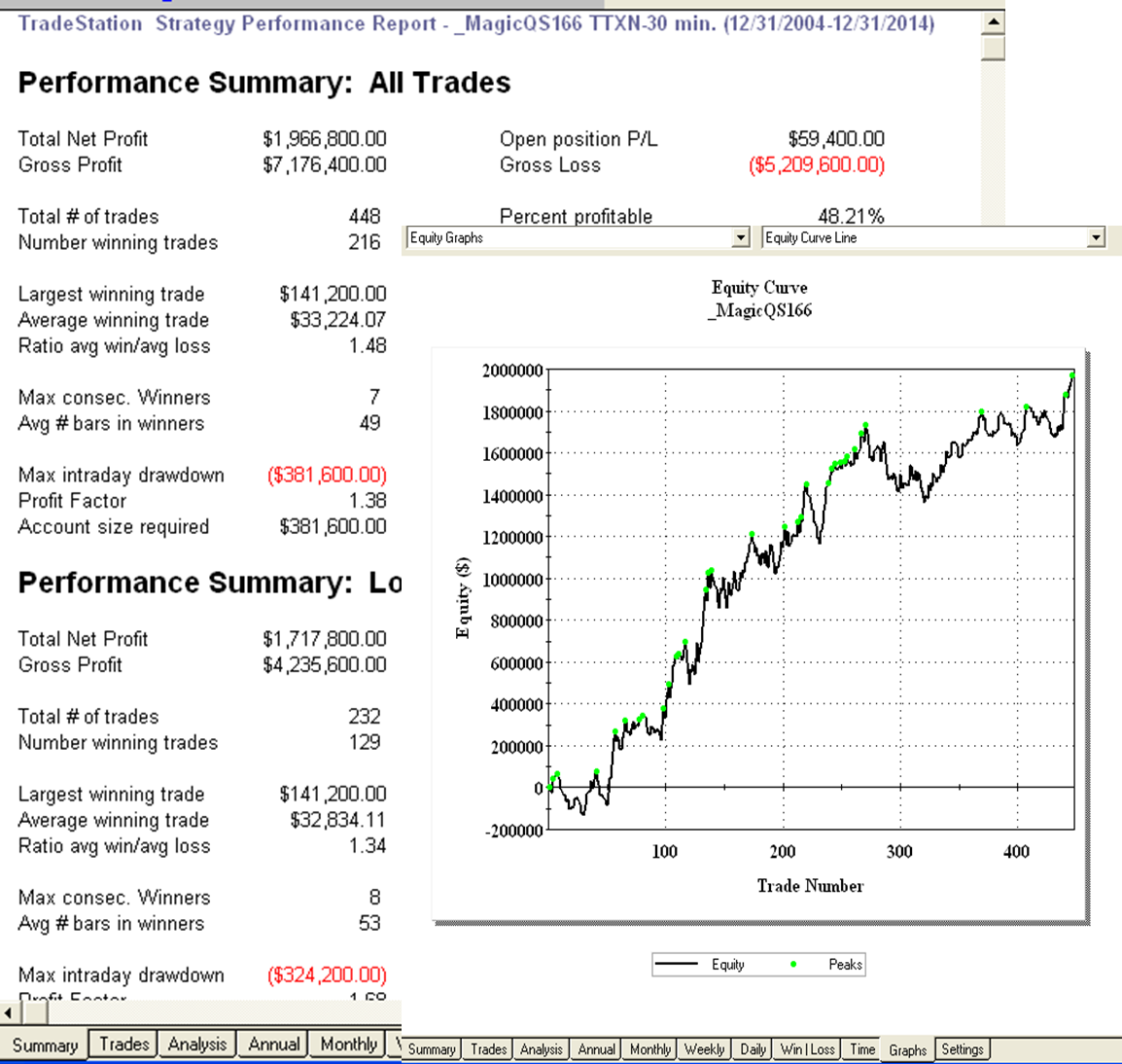## 2015年4月2日 星期四

### 開發商品的交易系統 - 基礎篇input:EntryType(2),ExitType(4);
vars: IsBalanceDay(False),MP(0),PF(0),PL(0),HLRange(100);
inputs: LenA(9),LenB(8),FracA(0.4),FracB(0.8),HighBar(11),LowBar(11);

MP = MarketPosition ;
if DAYofMonth(Date) > 14 and DAYofMonth(Date) < 22 and DAYofWeek(Date)= 3 then isBalanceDay = True else isBalanceDay =False ;

{計算導數}
AvgVal = Average(Close, LenA);
{第一次導數}
DerivMA1 = (AvgVal - AvgVal[LenA]) / LenA;
{第二次導數}
DerivMA2 = (DerivMA1 - DerivMA1) / 1;

{買賣環境建立}
Condition1 = DerivMA1 Crosses Below 0;
Condition2 = DerivMA1 Crosses Above 0;
Condition3 = DerivMA2 Crosses Below 0;
Condition4 = DerivMA2 Crosses Above 0;

{當第二次導數突破零軸時準備進場作多}
If Condition4 Then BuyFlag = True;

{當第二次導數跌破零軸時準備進場作空}
If Condition3 Then SellFlag = True;

{多方進場}
If MP <> 1 and Condition2 AND BuyFlag Then Begin
Buy Next Bar at Close + Average(Range,LenA)*FracA Stop;
End;

{空方進場}
If MP <> -1 and Condition1 AND SellFlag Then Begin
Sell Next Bar at Close - Average(Range,LenA)*FracB Stop;
SellFlag = False;
End;

{出場規則}
if ExitType = 1 then SetStopLoss(PL * BigPointValue) ;

if ExitType = 2 then Begin
SetStopLoss(PL * BigPointValue) ;
setProfitTarget(PF * BigPointValue) ;
end;

if ExitType = 3 then Begin
if MP > 0 and BarsSinceEntry = NBarL then ExitLong next bar at Market ;
if MP < 0 and BarsSinceEntry = NBarS then ExitShort next bar at Market ;
end;

if ExitType = 4 then Begin
SetStopLoss(PL * BigPointValue) ;
setProfitTarget(PF * BigPointValue) ;
if MP > 0 and BarsSinceEntry = NBarL then {Sell } ExitLong next bar at Market ;
if MP < 0 and BarsSinceEntry = NBarS then {Buy} ExitShort next bar at Market ;
end;

if IsBalanceDay or date = 1150224 then setExitonClose ;MagicQS166

#### 2 則留言:

1.Hi 版主大人，您好！

拜讀貴網站大作，獲益頗多。本人是初學者，有許多不懂之處，需要版主大人多多指教。

本人於 “開發商品的交易系統 - 基礎篇 ” 有不解之處（如下），請指點一二。謝謝！

{多方進場}
If MP <> 1 and Condition2 AND BuyFlag Then Begin
Buy Next Bar at Close + Average(Range,LenA)*FracA Stop;
End;

Buy Next Bar at Close + Average(Range,LenA)*FracA Stop; 為什麼要買在比 close + Avg(Range,LenA)*FracA 更高的價位呢？ 是為了確認次日價位有突破今日range 40%的向上趨勢嗎？
不能 Buy Next Bar at market?

新手上路，請多指教！

謝謝！

Nelson Liu

2.